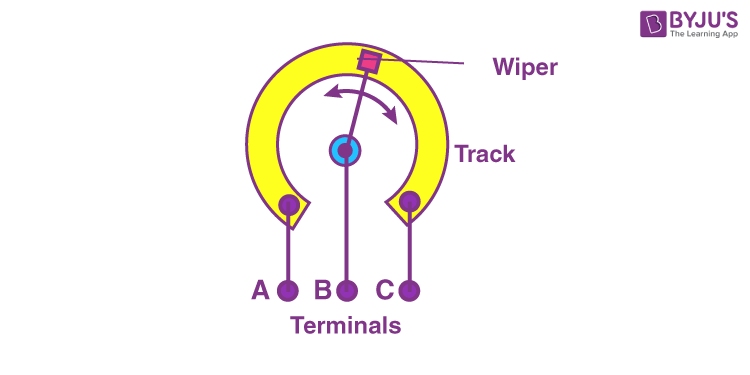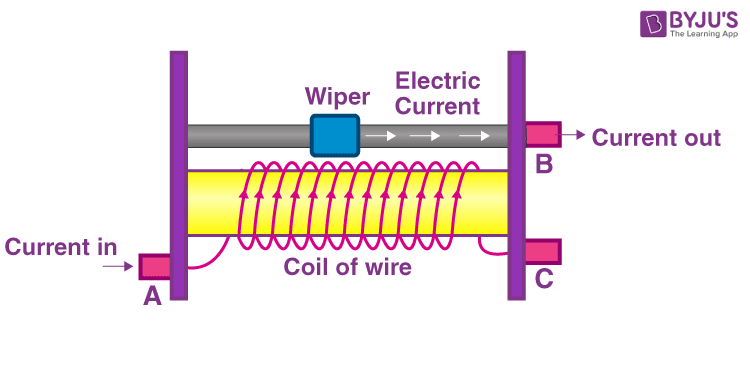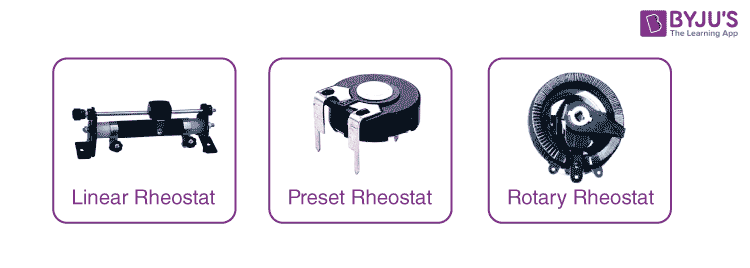# What is Rheostat

## Definition of Rheostat

A rheostat is defined as

A variable resistor which is used for controlling the flow of electric current either by increasing or decreasing the resistance.

The term rheostat was coined by the English scientist Sir Charles Wheatstone and is derived from the Greek word “rheos” and “statis” which means current controlling device.

### Rheostat SymbolThere are two rheostat symbols that are available, and they are the American standard and the international standard. The American standard symbol is represented with three terminals and zigzag lines while the international standard symbol is represented with a rectangular box with three terminals.

## Working of Rheostat

There are three main parameters of any electrical circuit, and they are:

• The voltage applied to the circuit
• Current through the circuit
• The resistance offered by the circuit

All three parameters are interdependent. For instance, to change the current flow, we have to either change the applied voltage or change the resistance. A rheostat is used in a circuit to change the resistance of the circuit so that the current changes. We also know that current and resistance are inversely proportional, that is current decreases with an increase in the resistance of the rheostat. It is also important to note that every rheostat comes with a resistance rating. If a rheostat has a rating of 500 kΩ, then the minimum resistance is 0 and the maximum resistance is 500 kΩ.

For any given rheostat, we can change its resistance. We know that resistance is dependent on three factors:

• Length
• Areas of cross-section
• Type

In order to change the resistance of the rheostat, the effective length needs to be changed with the help of sliding contact. The effective length is defined as the length between the fixed terminal and the position of the sliding terminal. As the effective length changes, the resistance of the rheostat changes.

## Construction of Rheostat

The construction of rheostat and potentiometer are similar. There are three terminals in the rheostat with two connections. The construction of rheostat is shown below:The three terminals of the rheostat are denoted as A, B and C. Either A and B terminals or B and C terminals are used. In the above figure, A and C are fixed and are connected towards the track which is known as the resistive element. Terminal B is uneven and is connected to the slider. As the wiper moves along the resistive element over the resistive path, the resistance of the rheostat changes. The rheostat’s resistive element is made with a loop of wire.

The resistance of the rheostat is dependent on the length of the resistive path.## Types of Rheostat

There are three types of rheostats and they are:

• Linear type
• Rotary type
• Preset type### Linear Rheostat

These are the rheostat types that includes a linear resistive lane such that the sliding terminal can be moved smoothly over the lane. There are two permanent terminals in which one of them is connected to the slider. The linear rheostat is also known as a cylindrical rheostat as its resistive element has a cylindrical view. These rheostats find applications in laboratories in research and teaching.

### Rotary Rheostat

These are the rheostat types that includes a rotating resistive lane. These rheostats are also known as the circular rheostat as the resistive element used appears to be a circle. The slider or the wiper moves linearly. The wiper is mounted on the shafts. These rheostats are commonly used in power applications.

### Preset Rheostat

These are the rheostat types that are used in the printed circuit board, and they are known as either trimmers or preset rheostat. They are commonly used in calibration circuits and are small.

## Applications of Rheostat

• The rheostat is used as a voltage divider.
• The rheostat also finds applications as a variable resistive load.
• In lighting circuits, the rheostats find applications as a dimming device.
• The study of electrical equipment and circuits become easy with the use of the rheostat as different current and voltage can be studied.
• The switching electronics are replaced by the rheostats.
• The rheostats find applications as power control devices in motor speed control, light intensity control and in heaters.

### Difference between Rheostat and Potentiometer

 Rheostat Potentiometer It is defined as an electrical instrument that is used for controlling the current by varying the resistance It is defined as an instrument that is used for measuring an electromotive force by altering the voltage It finds applications in motor control, light control, etc as it can handle higher current and voltage It finds electronic applications such as in electronic regulators and reference setters It is not necessary to use all the three terminals All the three terminals are used A rheostat cannot be used as a potentiometer A potentiometer can be used as a rheostat

## Frequently Asked Questions – FAQs

### Why is rheostat connected in series?

A rheostat is connected in series because the current flows through the path where the resistance is minimum.

### Which wire is used in rheostat?

The wire used in rheostat is Constantan. It is a copper-nickel alloy that is similar to manganin. It has a very low temperature coefficient of resistance, making its resistivity constant over a wide range of temperature.

### What is the difference between rheostat and resistance?

The difference between rheostat and resistance is that the rheostat is an electrical resistor with two terminals while the resistance is a parameter that can be varied continuously by moving the knob.

### Why does rheostat have three terminals?

The rheostat has three terminals as it is used as a potential divider, and it is used for eliminating the possibility of the open circuit due to the wiper movement.

### How to test a rheostat?

A rheostat is tested by measuring the resistance between any two fixed and variable points. The change in the resistance and the movement of the wiper from maximum to minimum should be proportional to each other. The value thus obtained should be similar to the rated value.